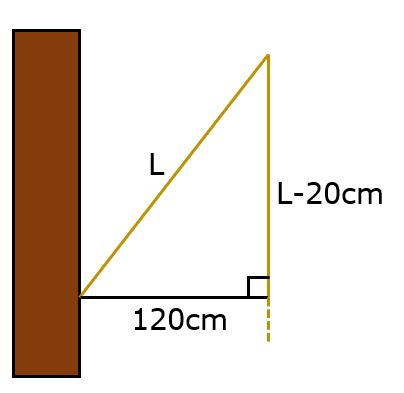# Tensar Summer Quiz - Answers Revealed!

by Andrew Lees, on 20-Aug-2020 04:33:39

A couple of weeks ago, Andrew Lees presented us with a new challenge in the form of the Tensar Summer Quiz! Following a much needed summer holiday, Andrew is back and has shared the answers below.

Please mark your own (remember to be honest) and let us know how you did in the comments below!

1. There are two ways to determine the time, by relative speed or algebraic equation:
Relative speed: the two boreholes approach each other at a relative speed of 50km/day and have covered a total distance of 12,730 km when they meet. So, the time taken equals 12,730km/(50km/day) = 254.6 days
Algebraic equation: let time taken equal t.
20t + 30t = 12,730
t = 254.6 days.

Meeting point:
Distance from UK: 20km/day * 254.6 days = 5,092km
In other words, 1,273km from the centre of the Earth on the UK side, where the heat and pressure would make drilling conditions challenging to say the least.

2. Using simultaneous equations, let H be the cost of a high-visibility vest and G the cost of a pair of goggles.
3H + 2G = 36
2H + 4G = 40
Rearranging the first equation to 2G = 36 – 3H
and substituting into the second equation:
2H + 72 – 6H = 40
H = 8.
So, 16 + 4G = 40
G = 6.
A pair of goggles costs £6.

3. There are 6 ways in which samples can be selected so that each of the three wells has contributed a sample to the test: ABC, ACB, BAC, BCA, CAB and CBA. Taking the first possibility (ABC), it can be calculated by noting there is a 1 in 6 chance of selecting Well A’s sample first, then a 2 in 5 probability of selecting one of Well B’s two samples from the remaining five samples, and then a 3 in 4 probability of selecting one of Well C’s three samples from the remaining four samples. So, the probability of that combination is: 1/6 x 2/5 x 3/4 =6/120 = 1/20 = 5%. Calculating the probability of any one of these 6 events is the same, (e.g. CBA is 3/6 x 2/5 x 1/4 = 5%). So, the probability of selecting three samples each from a different well is 6 times 5% =30%.

4. Let’s call the FPS’s age F and CECA’s C.
The first statement tells us that
5(C-16)=F-16,
which we can rearrange to give:
F = 5C-64.
The second statement tells us that
C-8 = 1/3 * (F-8),
which we can rearrange to give
3C=F+16.
Then we can substitute in for FPS’s age from the first rearranged expression and simplify to give
C=24.
Using the first rearranged expression we can find
F=56.
Therefore, the FPS and CECA were founded in 1964 and 1996 respectively.

5. The contractor should switch doors. Initially there is a 1/3 chance of choosing the right door, but when the head of procurement opens one of the doors revealing the potatoes and giving the contractor more information, there is a 2/3 chance that the third door is concealing the lucrative contract. This is known as the Monty Hall problem, named after the American game show host.

6. The front of the train passes through the tunnel in the time it takes for the train to travel the 100-metre length of the tunnel. Then the remaining 400-metre length of train needs to pass through, which requires the train to travel a further 400m, giving a total travel length of 500m to pass through the tunnel. So, the time taken is 0.5km/80km/h = 0.00625 hours or 22.5 seconds.

7. Trick question! Sorry, we couldn’t resist putting one in there! The question was worded to encourage the reader to add up the wrong numbers. In the end, the three people paid £27, £25 of which went to the pharmacist and £2 to the assistant. There is no missing pound!

8.Use Pythagoras' theorem
L2 = 1202 + (L-20)2
L2 = 14,400 + L2 – 40L + 400
40L = 14,800
L = 370cm

Interesting fact: the theorem attributed to Pythagoras was known and used by the Babylonians and Indians centuries before Pythagoras, although Pythagoras may not have known that. Historians still debate whether he himself made the discovery or whether it was colleagues or students at his school.
9. The van was losing 0.5% charge for every 1km travelled which, at a speed of 40km/h, is equivalent to losing 20% charge per hour. The battery was also losing 5% per hour for the air-conditioning, totalling 25% per hour. So, the van could go for four hours at 40km/h, making the total distance 160km.

10. For each real hour that passes, the clock shows 72 minutes has passed. So, the real time is a ratio of 60/72 of the time shown to have passed by the clock. Therefore, the real time that has passed during the exam after 3 hours shown by the clock equals 60/72 * 3 = 2.5 hours.

Well done to all those who took part!

Topics:Geogrid